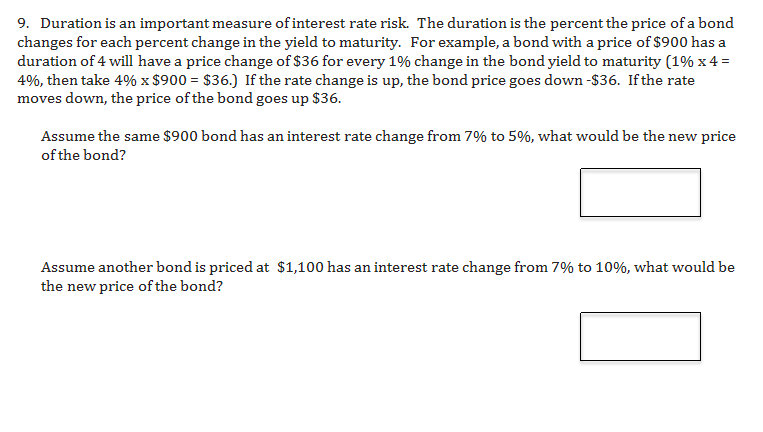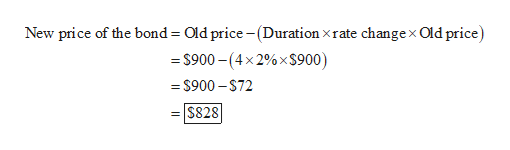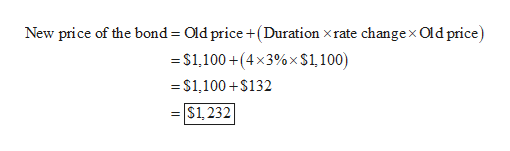# 9. Duration is an important measure of interest rate risk. The duration is the percent the price of a bondchanges for each percent change in the yield to maturity. For example, a bond with a price of \$900 has aduration of 4 will have a price change of \$36 for every 1% change in the bond yield to maturity (1% x 44%, then take 4% x \$900 \$36.) If the rate change is up, the bond price goes down -\$36. Ifthe ratemoves down, the price of the bond goes up \$36.Assume the same \$900 bond has an interest rate change from 7% to 5%, what would be the new priceof the bond?Assume another bond is priced at \$1,100 has an interest rate change from 7% to 10%, what would bethe new price of the bond?

Question
3 viewshelp_outlineImage Transcriptionclose9. Duration is an important measure of interest rate risk. The duration is the percent the price of a bond changes for each percent change in the yield to maturity. For example, a bond with a price of \$900 has a duration of 4 will have a price change of \$36 for every 1% change in the bond yield to maturity (1% x 4 4%, then take 4% x \$900 \$36.) If the rate change is up, the bond price goes down -\$36. Ifthe rate moves down, the price of the bond goes up \$36. Assume the same \$900 bond has an interest rate change from 7% to 5%, what would be the new price of the bond? Assume another bond is priced at \$1,100 has an interest rate change from 7% to 10%, what would be the new price of the bond? fullscreen
check_circle

Step 1

As per the given information, the percentage change in the bond\'s yield to maturity is 2% (7% - 5%) which is decreased from 7% to 5%. Hence, the new price of the bond is as follows:help_outlineImage TranscriptioncloseNew price of the bond Old price -(Duration xrate changex Old price) \$900-(4x2%x\$900) -\$900-\$72 =\$828 fullscreen
Step 2

Therefore, the new price of the bond is \$828.

Step 3

As per the given information, the percentage change in the bond's yield to maturity is 3% (10% ...help_outlineImage TranscriptioncloseNew price of the bond Old price +(Duration xrate change x Old price) =\$1,100+(4x3%x\$1, 100) =\$1,100+\$132 \$1,232 fullscreen

### Want to see the full answer?

See Solution

#### Want to see this answer and more?

Solutions are written by subject experts who are available 24/7. Questions are typically answered within 1 hour.*

See Solution
*Response times may vary by subject and question.
Tagged in